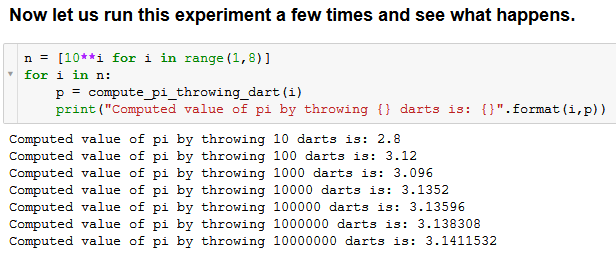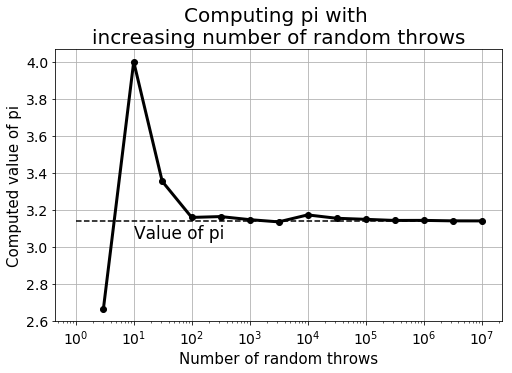# Mathematical programming - build up for advancing in data science

• 2019-04-17 08:03 AM
• 220

We show how, by simulating the random throw of a dart, you can compute the value of pi approximately. This is a small step towards building the habit of mathematical programming, which should be a key skill in the repertoire of a budding data scientist.

#### Introduction

The essence of mathematical programming is that you build a habit of coding up mathematical concepts, especially the ones involving a series of computational tasks in a systematic manner.

This kind of programming habit is extremely useful for a career in analytics and data science, where one is expected to encounter and make sense of a wide variety of numerical patterns every day. The ability of mathematical programming helps in rapid prototyping of quick numerical analyses, which are often the first steps in building a data model.

#### A few examples

So, what do I mean by mathematical programming? Isn’t a bunch of mathematical functions already built-in and optimized in various Python libraries like NumPy and SciPy?

Yes, but that should not stop you from coding up various numerical computation tasks from scratch and getting into the habit of mathematical programming.

Here are a few random examples,

As you can see, the examples can be made extremely interesting and close to real-life scenarios. This technique, therefore, also leads to the ability of writing code for discrete or stochastic simulations.

When you are browsing through some mathematical properties or ideas on the internet, do you feel the urge to quickly test the concept using a simple piece of code in your favorite programming language?

If yes, then congratulations! You have the ingrained habit of mathematical programming and it will take you far in your pursuit of a satisfactory data science career.

#### Why is mathematical programming a key skill for data science?

The practice of data science needs an extremely friendly relationship with numbers and numerical analyses. However, this does not mean memorization of complicated formula and equations.

A faculty of discovering patterns in numbers and an ability to quickly testing ideas by writing simple code go far for a budding data scientist.This is akin to an electronics engineer being fairly hands-on with laboratory-equipments and automation scripting to run those pieces of equipment to capture hidden patterns in the electrical signals.

Or, think of a young biologist who is proficient in creating cross-section samples of cells on a slide and quickly run automated tests under a microscope to gather data for testing her ideas.The point is that while the whole enterprise of data science may comprise of many disparate components — data wrangling, text processing, file handling, database processing, machine learning and statistical modeling, visualization, presentation, etc. — a quick experimentation with ideas often do not require much more than solid mathematical programming ability.

It is difficult to exactly pin-point all the necessary elements that are required to develop the skill of mathematical programming, but some of the common ones are,

• A habit of modularized programming,
• A clear idea about various randomization techniques
• Ability to read and understand the fundamental topics of linear algebra, calculus, and discrete mathematics,
• Familiarity with basic descriptive and inferential statistics,
• Rudimentary ideas about discrete and continuous optimization methods (such as linear programming)
• Basic proficiency with the core numerical libraries and functions in the language of choice, in which the data scientists wants to test her ideas

You can refer to this article which discusses what to learn in essential hands-on mathematics for data science.

In this article, we will illustrate the mathematical programming by discussing a very simple example, computing the approximate value of pi using a Monte Carlo method of throwing random darts at a board.

#### Computing pi by throwing (a lot of) darts

This is a fun method to compute the value of pi by simulating the random process of throwing darts at a board. It does not use any sophisticated mathematical analysis or formula but tries to compute the approximate value of pi from the emulation of a purely physical (but stochastic) process.

This technique falls under the banner of Monte Carlo method, whose basic concept is to emulate a random process, which, when repeated a large number of times, gives rise to the approximation of some mathematical quantity of interest.

Imagine a square dartboard.

Then, the dartboard with a circle drawn inside it touching all its sides.

And then, you throw darts at it. Randomly. That means some fall inside the circle, some outside. But assume that no dart falls outside the board.At the end of your dart throwing session, you count the fraction of darts that fell inside the circle of the total number of darts thrown. Multiply that number by 4.

The resulting number should be pi. Or, a close approximation if you had thrown a lot of darts.

#### What’s the idea?

The idea is extremely simple. If you throw a large number of darts, then the probability of a dart falling inside the circle is just the ratio of the area of the circle to that of the area of the square board. With the help of basic mathematics, you can show that this ratio turns out to be pi/4. So, to get pi, you just multiply that number by 4.

The key here is to simulate the throwing of a lot of darts so as to make the fraction (of the darts that fall inside the circle) equal to the probability, an assertion valid only in the limit of a large number of trials of this random event. This comes from the law of large number or the frequentist definition of probability.

#### Python code

A Jupyter notebook illustrating the Python code is given here in my Github repo. Please feel free to copy or fork. The steps are simple.

First, create a function to simulate the random throw of a dart.

``````def throw_dart():
"""
Simulates the randon throw of a dart. It can land anywhere in the square (uniformly randomly)
"""
# Center point
x,y = 0,0
# Side of the square
a = 2

# Random final landing position of the dart between -a/2 and +a/2 around the center point
position_x = x+a/2*(-1+2*random.random())
position_y = y+a/2*(-1+2*random.random())

return (position_x,position_y)
``````

compute_pi_dart_1.py

Then, write a function to determine if a dart, given its landing coordinates, falls inside the circle,

``````def is_within_circle(x,y):
"""
Given the landing coordinate of a dart, determines if it fell inside the circle
"""
# Side of the square
a = 2

distance_from_center = sqrt(x**2+y**2)

if distance_from_center < a/2:
return True
else:
return False
``````

compute_pi_dart_2.py

Finally, write a function to simulate a large number of dart throwing and calculate the value of pi from the cumulative result.

``````def compute_pi_throwing_dart(n_throws):
"""
Computes pi by throwing a bunch of darts at the square
"""
n_throws = n_throws
count_inside_circle=0
for i in range(n_throws):
r1,r2=throw_dart()
if is_within_circle(r1,r2):
count_inside_circle+=1

result = 4*(count_inside_circle/n_throws)

return result
``````

compute_pi_dart_3.py

But the programming must not stop there. We must test how good the approximation is and how it changes with the number of random throws. As with any Monte Carlo experiment, we expect the approximation to get better with a higher number of experiments.

This is the core of data science and analytics. It is not enough to write a function which prints the expected output and stop there. The essential programming may be done but the scientific experiment does not stop there without further exploration and testing of the hypothesis.We can see that a large number of random throws can be repeated a few times to calculate an average and get a better approximation.

``````n = 5000000
sum=0
for i in range(20):
p=compute_pi_throwing_dart(n)
sum+=p
print("Experiment number {} done. Computed value: {}".format(i+1,p))
print("-"*75)
pi_computed = round(sum/20,4)
print("Average value from 20 experiments:",pi_computed)
``````

compute_pi_dart_4.py

#### Simple code, rich ideas

The theory and code behind this technique seem extremely simple. However, behind the facade of this simple exercise, some pretty interesting ideas are hidden.

Functional programming approach: The description of the technique can be coded using a monolith code block. However, we show how the tasks should be partitioned into simple functions mimicking real human actions —

• throwing a dart,
• examining the landing coordinate of the dart and determining whether it landed inside the circle,
• repeating this process an arbitrary number of times

To write high-quality code for large programs, it is instructive to use this kind of modularized programming style.

Emergent behavior: Nowhere in this code, any formula involving pi or properties of a circle was used. Somehow, the value of pi emerges from the collective action of throwing a bunch of darts randomly at a board and calculating a fraction. This is an example of emergent behavior in which a mathematical pattern emerges from a set of a large number of repeated experiments of a similar kind through their mutual interaction.

Frequentist definition of probability: There are two broad categories of the definition of probability and two fiercely rival camps — frequentists and Bayesians. It is easy to think as a frequentist and define probability as a frequency (as a fraction of the total number of random trials) of an event. In this coding exercise, we could see how this particular notion of probability emerges from repeating random trials a large number of times.

Stochastic simulation: The core function of throwing dart uses a random generator at its heart. Now, a computer-generated random number is not truly random, but for all practical purpose, it can be assumed to be one. In this programming exercise, we used a uniform random generator function from the `random` module of Python. Use of this kind of randomization method is at the heart of stochastic simulation, which is a powerful method used in the practice of data science.

Testing the assertion by repeated simulations and visualization: Often, in data science, we deal with stochastic processes and probabilistic models, which must be tested based on a large number of simulations/experiments. Therefore, it is imperative to think in those asymptotic terms and test the validity of the data model or the scientific assertion in a statistically sound manner.#### Summary (and a challenge for the reader)

We demonstrate what it means to develop a habit of mathematical programming. Essentially, it is thinking in terms of programming to test out the mathematical properties or data patterns that you are developing in your mind. This simple habit can aid in the development of good practices for an upcoming data scientist.

An example was demonstrated using simple geometric identities, concepts of stochastic simulation, and frequentist definition of probability.

If you are looking for more challenge,

can you compute pi by simulating a random walk event?

If you want to fork the code for this fun exercise, please fork this repo.